# AP Physics C Electricity : Understanding Electromagnetic Induction

## Example Questions

### Example Question #1 : Understanding Electromagnetic Induction

A metal ring is placed in a uniform magnetic field perpendicular to the plane of the ring. An emf of magnitude 15V is induced around the ring by increasing the field through it from zero to 5mT at a constant rate. If the area enclosed by the ring is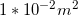, what is the time interval over which the field is increased?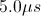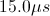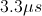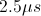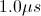Explanation:

Relevant equations: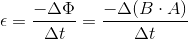Given: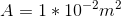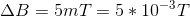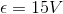Plug in (disregarding direction of emf):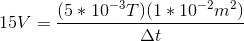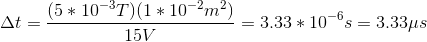### All AP Physics C Electricity Resources